Year 6 maths practise

Have your kid go over some of the important ideas covered in year 6 maths. After she's mastered these concepts, she'll be ready to tackle maths in intermediate.

Click on a worksheet in the set below to see more info or download the PDF.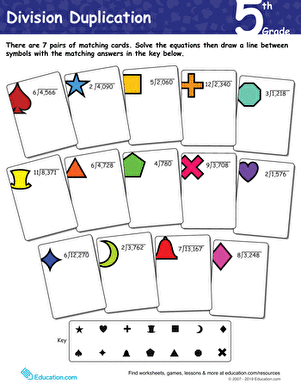Division Duplication: year 6

Long division may be tough to master, and year six students can use this maths worksheet to practise this important skill.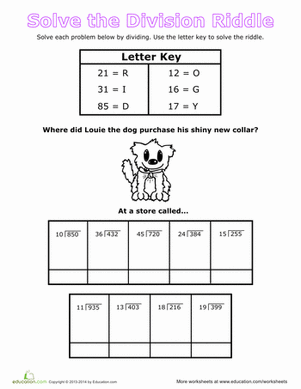Solve a Division Riddle

Fifth graders practise multi-digit division as they work on cracking the riddle.Dividing Decimals

Children learn how to divide multi-digit decimal numbers in this supplemental maths worksheet.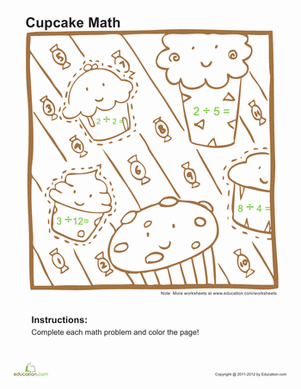Division Coloring Page

Give your child a few division practise problems with a cute, cupcake coloring page! She'll work on some simple division facts, and a few tougher equations.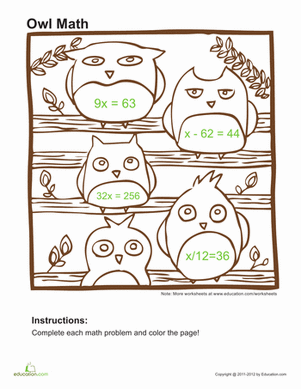Solve for X

For beginning algebra students, take a break from boring textbook equations and get some practise solving for x with a colorful worksheet!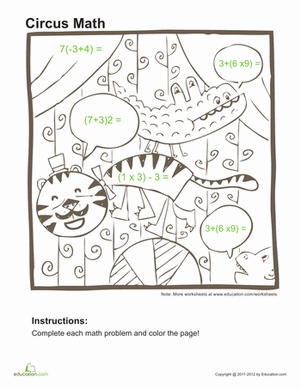Order of Operations practise #1

Get ready for algebra with some basic order of operations practise problems. After your child solves the equations, he'll have a picture to colour in!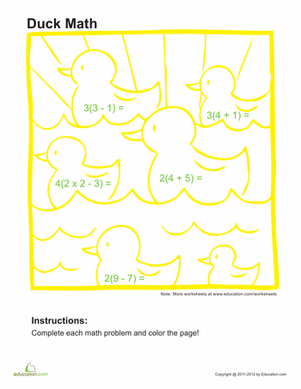Order of Operations practise #2

In this order of operations practise sheet, maths and coloring go hand in hand! Your child will get to colour a cute picture as she completes the equations.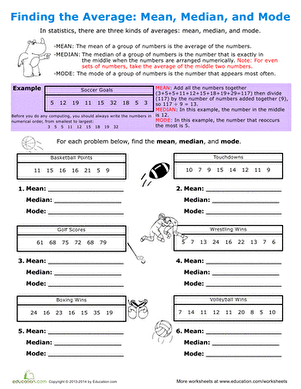Finding the Average: Mean, Median, and Mode

Help the concept of averages sink in for your fifth grader with this worksheet all about finding the mean, median, and mode.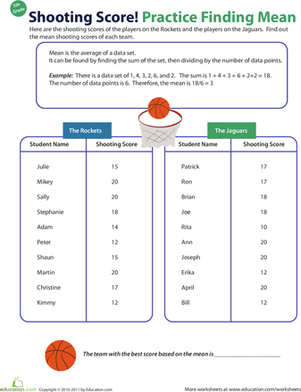Determine Mean

Which team played better? Let your fifth grader practise finding averages by determining the mean shooting scores of each team.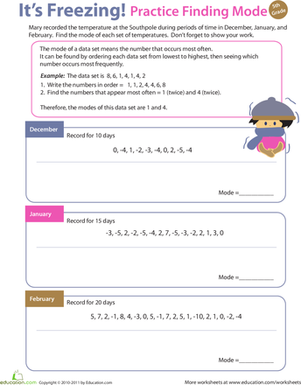It's Freezing! practise Finding Mode

This colorful worksheet will get your fifth grader practising with mode: the number in a set of data that occurs most often.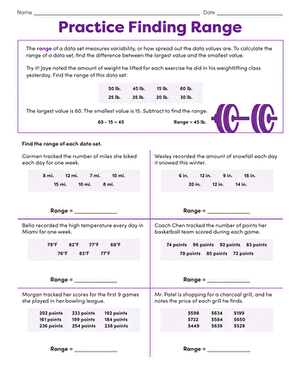What's the Range?

Use this colorful worksheet to teach your fifth grader range. It'll get him familiar with basic statistics.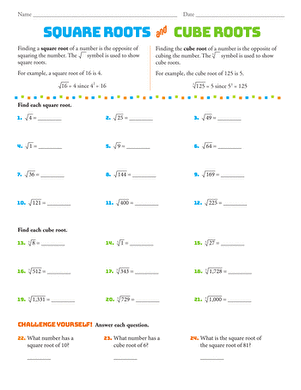Building Exponents: Squares, Cubes, and Roots

Kids review squared and cubed numbers, then try their hand at finding the square root or cube root of a number in this beginning algebra worksheet.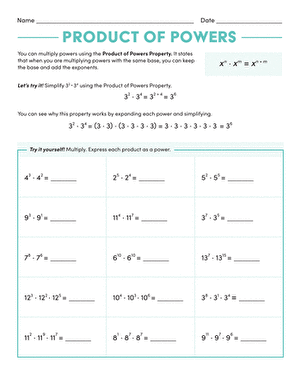Multiplying with Exponents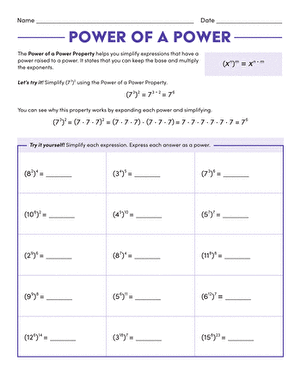Multiplying Numbers with Exponents

Raising an exponential expression to a power isn't half as difficult as it sounds. Challenge your fifth grader to give it a try with help from this worksheet.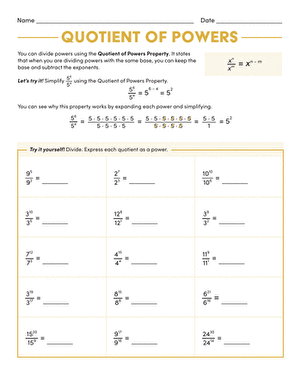Power Play: Dividing with Exponents

Don't let your fifth grader despair--when the base numbers are the same, dividing with exponents isn't tricky at all thanks to a helpful formula.Geometry Review: Angles and Polygons

Fifth graders review types of angles, triangles, and polygons in this handy geometry review.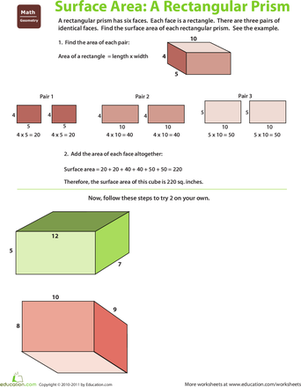Find the Surface Area: Rectangular Prism

Is your fifth grader ready to dig a little deeper into geometry? This exercise shows her how to find the surface area of a rectangular prism.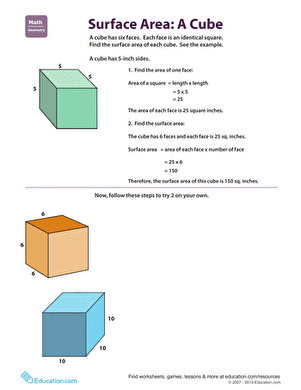Find the Surface Area: Cube

Once your fifth grader has mastered the basics, it's time to start finding the surface areas of 3-D shapes--like cubes.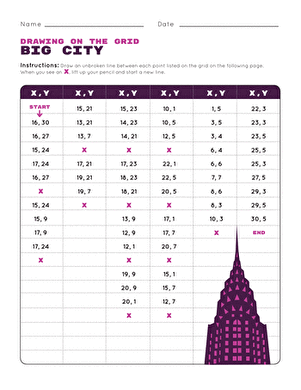Mystery Picture Graph #1

Have a little fun with graphing practise in the big city! maths students will love plotting points on this coordinate grid as they see a mystery picture emerge.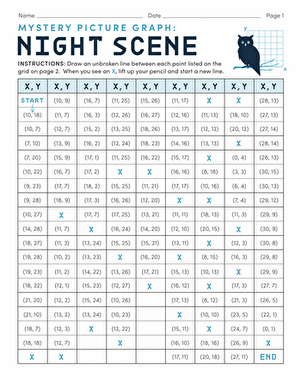Mystery Picture Graph #2

This mysterious maths sheet is full of fun for geometry students! They'll plot these points on a coordinate grid to reveal a picture.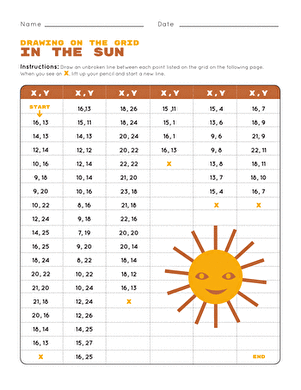Mystery Picture Graph #3

Can you discover the mystery picture? Make maths fun with this coordinate grid activity, where your child will reveal a hidden picture with every point he plots!How to Order Decimals

Sort out decimals on a number line in this quick drill worksheet about how to order decimals.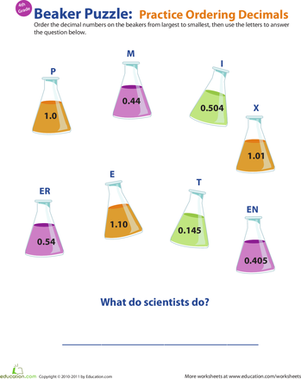Ordering Decimals practise

Does your fourth grader need help with ordering decimals? Bolster his decimal understanding with this beaker-booked worksheet.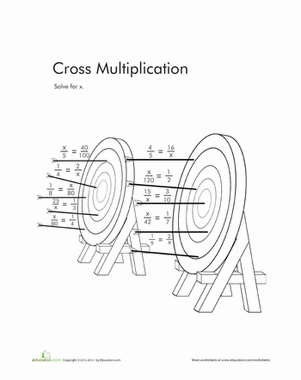Cross-Multiplication

Print off this sheet of cross multiplication exercises to sharpen your child's cross multiplication skills.

Want to download this whole set as a single PDF?

Create new collection

0

New Collection>

0Items

What could we do to improve Education.com?

Please note: Use the Contact Us link at the bottom of our website for account-specific questions or issues.

What would make you love Education.com?